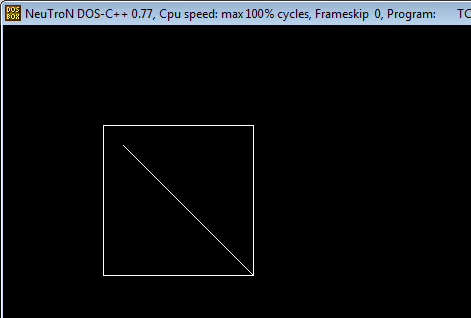# Liang Barsky Line Clipping Algorithm in C and C++

Here you will learn about liang barsky line clipping algorithm in C and C++.

This Algorithm was developed by Liang and Barsky. It is used for line clipping as it is more efficient than Cyrus Beck algorithm and Cohen Sutherland algorithm because it uses more efficient parametric equations to clip the given line.

These parametric equations are given as:

x = x1 + tdx

y = y1 + tdy, 0 <= t <= 1

Where dx = x2 – x1 & dy = y2 – y1

Liang Barsky line clipping algorithm uses 4 inequalities with 2 parameters p & q which are defined in the algorithm below.

### Algorithm

1. Read 2 endpoints of line as p1 (x1, y1) & p2 (x2, y2).

2. Read 2 corners (left-top & right-bottom) of the clipping window as (xwmin, ywmin, xwmax, ywmax).

3. Calculate values of parameters pi and qi for i = 1, 2, 3, 4 such that

p1 = -dx, q1 = x1 – xwmin

p2 = dx, q2 = xwmax – x1

p3 = -dy, q3 = y1 – ywmin

p4 = dy, q4 = ywmax – y1

4. if pi = 0 then line is parallel to ith boundary

if qi < 0 then line is completely outside boundary so discard line

else, check whether line is horizontal or vertical and then check the line endpoints with the corresponding boundaries.

5. Initialize t1 & t2 as

t1 = 0 & t2 = 1

6. Calculate values for qi/pi for i = 1, 2, 3, 4.

7. Select values of qi/pi where pi < 0 and assign maximum out of them as t1.

8. Select values of qi/pi where pi > 0 and assign minimum out of them as t2.

9. if (t1 < t2)
{
xx1 = x1 + t1dx

xx2 = x1 + t2dx

yy1 = y1 + t1dy

yy2 = y1 + t2dy

line (xx1, yy1, xx2, yy2)
}

10. Stop.

1. More efficient than other algorithms as line intersection with boundaries calculations are reduced.

2. Intersections of line are computed only once.

## Program for Liang Barsky Line Clipping Algorithm in C and C++

### C++ Program

OutputAuthor Bio:

I am Rahul Maheshwari from India currently pursuing engineering degree in Computer Science. I am passionate about programming and loves to code as much as I can and likes to help people to become better in programming.

1.